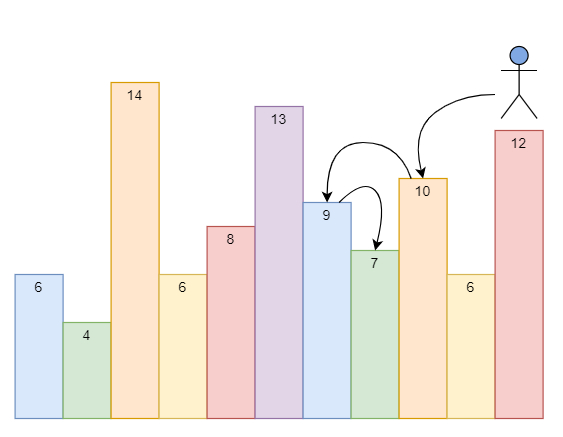# Jump Game V in C++

Suppose we have an array of integers called arr and an integer d. In one step we can jump from index i to −

• i + x where: i + x < n and x in range 1 to d.

• i - x where: i - x >= 0 and x in range 1 to d.

Here n is the size of array. In addition, we can only jump from index i to index j when arr[i] > arr[j] and arr[i] > arr[k] for all indices k between i and j. We can choose any index of the array and start the jumping. We have to find the maximum number of indices we can visit.

So, if the input is like d = 2 and heights are likethen the output will be 4, we can start at index 10. we can jump from index 10 --> 8 --> 6 --> 7 as shown. So if we start at index 6 we can only jump to index 7. We cannot jump to index 5 because 13 > 9. We cannot jump to index 4 because index 5 is between index 4 and 6 and 13 > 9. And also, we cannot jump from index 3 to index 2 or index 1.

To solve this, we will follow these steps −

• Define an array dp

• Define a function solve(), this will take an array arr, idx, d,

• if dp[idx] is not equal to -1, then −

• return dp[idx]

• ret := 1

• n := size of arr

• for initialize i := idx + 1, when i < n, update (increase i by 1), do −

• if i > idx + d, then −

• Come out from the loop

• if arr[i] >= arr[idx], then −

• Come out from the loop

• ret := maximum of ret and 1 + solve(arr, i, d)

• for initialize i := idx - 1, when i >= 0, update (decrease i by 1), do −

• if i < idx - d, then −

• Come out from the loop

• if arr[i] >= arr[idx], then −

• Come out from the loop

• ret := maximum of ret and 1 + solve(arr, i, d)

• dp[idx] := ret

• return ret

• From the main method do the following −

• n := size of arr

• dp := Define an array of size n and fill this with -1

• ret := 1

• for initialize i := 0, when i < n, update (increase i by 1), do −

• ret := maximum of ret and solve(arr, i, d)

• return ret

Let us see the following implementation to get better understanding −

## Example

Live Demo

#include <bits/stdc++.h>
using namespace std;
class Solution {
public:
vector<int> dp;
int solve(vector <int>& arr, int idx, int d){
if (dp[idx] != -1)
return dp[idx];
int ret = 1;
int n = arr.size();
for (int i = idx + 1; i < n; i++) {
if (i > idx + d)
break;
if (arr[i] >= arr[idx])
break;
ret = max(ret, 1 + solve(arr, i, d));
}
for (int i = idx - 1; i >= 0; i--) {
if (i < idx - d)
break;
if (arr[i] >= arr[idx])
break;
ret = max(ret, 1 + solve(arr, i, d));
}
return dp[idx] = ret;
}
int maxJumps(vector<int>& arr, int d) {
int n = arr.size();
dp = vector<int>(n, -1);
int ret = 1;
for (int i = 0; i < n; i++) {
ret = max(ret, solve(arr, i, d));
}
return ret;
}
};
main(){
Solution ob;
vector<int> v = {6,4,14,6,8,13,9,7,10,6,12};
cout << (ob.maxJumps(v, 2));
}

## Input

{6,4,14,6,8,13,9,7,10,6,12}, 2

## Output

4